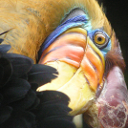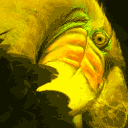Related Articles
Image compression using K-means clustering
• Last Updated : 13 May, 2019

Prerequisite: K-means clustering

The internet is filled with huge amounts of data in the form of images. People upload millions of pictures every day on social media sites such as Instagram, Facebook and cloud storage platforms such as google drive, etc. With such large amounts of data, image compression techniques become important to compress the images and reduce storage space.

In this article, we will look at image compression using K-means clustering algorithm which is an unsupervised learning algorithm.

An image is made up of several intensity values known as Pixels. In a colored image, each pixel is of 3 bytes containing RGB (Red-Blue-Green) values having Red intensity value, then Blue and then Green intensity value for each pixel.

Approach:
K-means clustering will group similar colors together into ‘k’ clusters (say k=64) of different colors (RGB values). Therefore, each cluster centroid is the representative of the color vector in RGB color space of its respective cluster. Now, these ‘k’ cluster centroids will replace all the color vectors in their respective clusters. Thus, we need to only store the label for each pixel which tells the cluster to which this pixel belongs. Additionally, we keep the record of color vectors of each cluster center.

Libraries needed –

-> Numpy library: `sudo pip3 install numpy`.
-> Matplotlib library: `sudo pip3 install matplotlib`.
-> scipy library: `sudo pip3 install scipy`

Below is the Python implementation :

 `import` `numpy as np``import` `matplotlib.pyplot as plt``import` `matplotlib.image as img``# from scipy.io import loadmat``from` `scipy ``import` `misc`` ` `def` `read_image():``     ` `    ``# loading the png image as a 3d matrix ``    ``img ``=` `misc.imread(``'bird_small.png'``) `` ` `    ``# uncomment the below code to view the loaded image``    ``# plt.imshow(A) # plotting the image``    ``# plt.show() ``     ` `    ``# scaling it so that the values are small``    ``img ``=` `img ``/` `255` ` ` `    ``return` `img`` ` `def` `initialize_means(img, clusters):``     ` `    ``# reshaping it or flattening it into a 2d matrix``    ``points ``=` `np.reshape(img, (img.shape[``0``] ``*` `img.shape[``1``],``                                             ``img.shape[``2``])) ``    ``m, n ``=` `points.shape`` ` `    ``# clusters is the number of clusters``    ``# or the number of colors that we choose.``     ` `    ``# means is the array of assumed means or centroids. ``    ``means ``=` `np.zeros((clusters, n)) `` ` `    ``# random initialization of means. ``    ``for` `i ``in` `range``(clusters):``        ``rand1 ``=` `int``(np.random.random(``1``)``*``10``)``        ``rand2 ``=` `int``(np.random.random(``1``)``*``8``)``        ``means[i, ``0``] ``=` `points[rand1, ``0``]``        ``means[i, ``1``] ``=` `points[rand2, ``1``]`` ` `    ``return` `points, means`` ` ` ` `# Function to measure the euclidean``# distance (distance formula)``def` `distance(x1, y1, x2, y2):``     ` `    ``dist ``=` `np.square(x1 ``-` `x2) ``+` `np.square(y1 ``-` `y2)``    ``dist ``=` `np.sqrt(dist)`` ` `    ``return` `dist`` ` ` ` `def` `k_means(points, means, clusters):`` ` `    ``iterations ``=` `10` `# the number of iterations ``    ``m, n ``=` `points.shape``     ` `    ``# these are the index values that``    ``# correspond to the cluster to``    ``# which each pixel belongs to.``    ``index ``=` `np.zeros(m) `` ` `    ``# k-means algorithm.``    ``while``(iterations > ``0``):`` ` `        ``for` `j ``in` `range``(``len``(points)):``             ` `            ``# initialize minimum value to a large value``            ``minv ``=` `1000``            ``temp ``=` `None``             ` `            ``for` `k ``in` `range``(clusters):``                 ` `                ``x1 ``=` `points[j, ``0``]``                ``y1 ``=` `points[j, ``1``]``                ``x2 ``=` `means[k, ``0``]``                ``y2 ``=` `means[k, ``1``]``                 ` `                ``if``(distance(x1, y1, x2, y2) < minv):         ``                    ``minv ``=` `distance(x1, y1, x2, y2)``                    ``temp ``=` `k``                    ``index[j] ``=` `k ``         ` `        ``for` `k ``in` `range``(clusters):``             ` `            ``sumx ``=` `0``            ``sumy ``=` `0``            ``count ``=` `0``             ` `            ``for` `j ``in` `range``(``len``(points)):``                 ` `                ``if``(index[j] ``=``=` `k):``                    ``sumx ``+``=` `points[j, ``0``]``                    ``sumy ``+``=` `points[j, ``1``] ``                    ``count ``+``=` `1``             ` `            ``if``(count ``=``=` `0``):``                ``count ``=` `1`    `             ` `            ``means[k, ``0``] ``=` `float``(sumx ``/` `count)``            ``means[k, ``1``] ``=` `float``(sumy ``/` `count)     ``             ` `        ``iterations ``-``=` `1`` ` `    ``return` `means, index`` ` ` ` `def` `compress_image(means, index, img):`` ` `    ``# recovering the compressed image by``    ``# assigning each pixel to its corresponding centroid.``    ``centroid ``=` `np.array(means)``    ``recovered ``=` `centroid[index.astype(``int``), :]``     ` `    ``# getting back the 3d matrix (row, col, rgb(3))``    ``recovered ``=` `np.reshape(recovered, (img.shape[``0``], img.shape[``1``],``                                                     ``img.shape[``2``]))`` ` `    ``# plotting the compressed image.``    ``plt.imshow(recovered)``    ``plt.show()`` ` `    ``# saving the compressed image.``    ``misc.imsave(``'compressed_'` `+` `str``(clusters) ``+``                        ``'_colors.png'``, recovered)`` ` ` ` `# Driver Code``if` `__name__ ``=``=` `'__main__'``:`` ` `    ``img ``=` `read_image()`` ` `    ``clusters ``=` `16``    ``clusters ``=` `int``(``input``(``'Enter the number of colors in the compressed image. default = 16\n'``))`` ` `    ``points, means ``=` `initialize_means(img, clusters)``    ``means, index ``=` `k_means(points, means, clusters)``    ``compress_image(means, index, img)`

Input Image:Output :My Personal Notes arrow_drop_up